How Cheenta works to ensure student success?
Explore the Back-Story

# Sum of two digit numbers | PRMO-2016 | Problem 7Try this beautiful problem from Algebra based on Sum of two digit numbers from PRMO 2016.

## Sum of two digit numbers | PRMO | Problem 7

Let s(n) and p(n) denote the sum of all digits of n and the products of all the digits of n(when written in decimal form),respectively.Find the sum of all two digits natural numbers n such that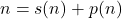••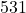•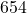### Key Concepts

Algebra

number system

Answer:PRMO-2016, Problem 7

Pre College Mathematics

## Try with Hints

Let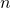is a number of two digits ,ten's place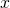and unit place is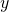.so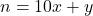.given that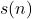= sum of all digits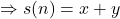and=product of all digits=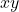now the given condition isCan you now finish the problem ..........

Fromcondition we have,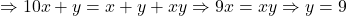and the value ofbe any digit....

Can you finish the problem........

Therefore all two digits numbers are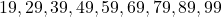and sum=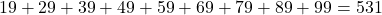## Subscribe to Cheenta at Youtube

Try this beautiful problem from Algebra based on Sum of two digit numbers from PRMO 2016.

## Sum of two digit numbers | PRMO | Problem 7

Let s(n) and p(n) denote the sum of all digits of n and the products of all the digits of n(when written in decimal form),respectively.Find the sum of all two digits natural numbers n such that•••### Key Concepts

Algebra

number system

Answer:PRMO-2016, Problem 7

Pre College Mathematics

## Try with Hints

Letis a number of two digits ,ten's placeand unit place is.so.given that= sum of all digitsand=product of all digits=now the given condition isCan you now finish the problem ..........

Fromcondition we have,and the value ofbe any digit....

Can you finish the problem........

Therefore all two digits numbers areand sum=## Subscribe to Cheenta at Youtube

This site uses Akismet to reduce spam. Learn how your comment data is processed.

### Knowledge Partner